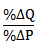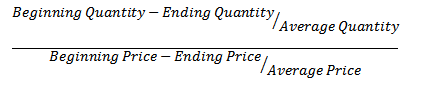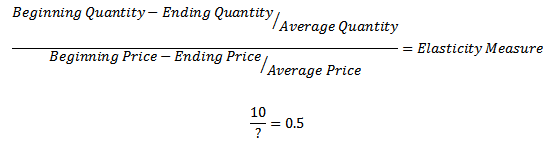FreeEconHelp.com, Learning Economics... Solved!: price elasticity of demand
Showing posts with label price elasticity of demand. Show all posts
Showing posts with label price elasticity of demand. Show all posts

## Using the price elasticity of demand to solve for a percent change in quantity

11:29
Figuring out how to calculate the price elasticity of demand can be one of the most abstract concepts for students. However, it is a very useful measure, and businesses and the government are very interested in price elasticity of demand measures for all sorts of goods. Imagine, if netflicks knew the price elasticity of demand for their product, they would have never raised their price as they did, and the following fallout could have been avoid.

For this post on economics, we consider the following question relating to the price elasticity of demand:

## Monopoly and monopolistic revenues, equations, elasticities, and price discrimination

11:55
This post is going over a question recently received:

You have been assigned the task of helping the Midland Milk Marketing (MMM),
the sole marketing agency for milk produced in the Island State of Midland. In the past the producers have been marketing their milk as a homogeneous commodity to all processors of milk. You have studied the market extensively and realize that the market can be segmented into two separate units:

(1) the market for fluid milk (milk for drinking) and (2) the market for processing milk (for manufacture of cheese, etc.). Your preliminary analysis has generated the following demand curves for the two separate markets.

Fluid milk market:

## Business revenue and elasticities

11:59
SUMMARY: If price elasticity of a good is inelastic (<1), then a firm can increase revenue by raising price.  If the price elasticity of a good is elastic (>1), then a firm can increase revenue by lowering price.

Understanding what the price elasticity of demand is can be confusing.  You may also be asking yourself why you need to know it in the first place.  But understanding what the price elasticity of demand measure is for a specific good can be important to business and marketing people when they are trying to maximize their revenue.  Take for example the common problem of choosing the price at which you want to sell a good.

Begin with a firm that already has its price set.  It knows what the price elasticity of demand is, and has to make a judgement call on whether to a price increase or decrease will increase their revenue.

First we have to remember the equation for the price elasticity of demand:
Percent change in quantity/percent change in price = price elasticity of demand.

So we know that if the percent change in quantity is greater than the percent change in price, then we

## Using the midpoint formula to solve elasticity questions in economics

16:20

Most economics classes will require you to use the midpoint formula in order to solve elasticity questions.  They require this because a percent change in a given problem could be different depending on whether the price is increasing, or falling.  Check out the example below for a price change from \$5 to \$10:

If the price increases to \$10, then we have (\$10-\$5)/\$5, which gives us \$5/\$5, or 100%

However if the price decreases we have (\$5-\$10)/\$10, which gives us -\$5/\$10, or -50%.

So depending on whether it is a price increase or decrease, then we will see different percentage

## What does an elasticity measure of -.2 mean?

18:44
What do elasticity measures tell us?

Well it depends on which elasticity we are looking at.  If we are considering the price elasticity of demand, shown right, then having an elasticity measure of -.2 means that as price goes up by some percent change, then quantity goes down by that percent change multiplied by -.2.

This is a good result, because it is saying that as the price goes up, we demand less of that good.  How much less of the good, only -.2, which means that the good is INELASTIC.  As long as the elasticity measure is below 1 (or above -1), then the quantity changes at a relatively lower amount than price, so we call that inelastic.  See the post on elasticities for more of an explanation.

What if the price elasticity of supply was -.2?  Then we would be saying if price

## How to solve elasticities problems in economics

15:36
Calculating elasticities is actually very easy.  You just need to know several of the values, then plug them into their proper place.The first equation is for the price elasticity of demand.  In order to calculate this, we need the beginning and ending price, and the beginning and ending quantity.  By beginning and ending, I mean before and after the price/quantity change.Here just plug in the values given to their appropriate spot.  Some students get confused about which price/quantity goes first, but actually it does not matter as long as you are consistent.  Try it with some example values and you will see that subtracting ending from beginning or vice verse has no impact on the final answer.

If you get a question that gives you the elasticity measurement and wants you to solve for one of the price or quantity levels, this is also easy to solve for given some algebraic manipulation.Divide both sides by 0.5 and multiply both sides by ? to end up with 20=?, so we know that the percent change in price had to be 20, in order to get the elasticity measure of 0.5.

Another example:
Suppose the price elasticity of demand coefficient for the product of a firm is 2,0 (ignore negative sign) the firm decides to increase the price of its product from 600 to 612. if the firm is currently selling 1500 units per month, how many will be sold after the price increase?

To get this number we have to figure out what the % change in price is first: 612-600/600 =  .02% change.  Now we know that the elasticity of demand is 2.0, so we can multiply our elasticity measure by the % change in price to get our % change in quantity, remember:

% change in quantity / % change in price = elasticity

we can rearrange this to get:

elasticity * % change in price = % change in quantity

or for our problem:

2 * 0.02 = 0.04

We now have to change the quantity by -4 percent, or mutiply 1500 by 96% (100-4) to get:
1500*.96=1440

So the quantity sold after the price increase goes down by 60.  Also note that total revenue has declined.  Click here for more info on the relationship between revenue and elasticities.